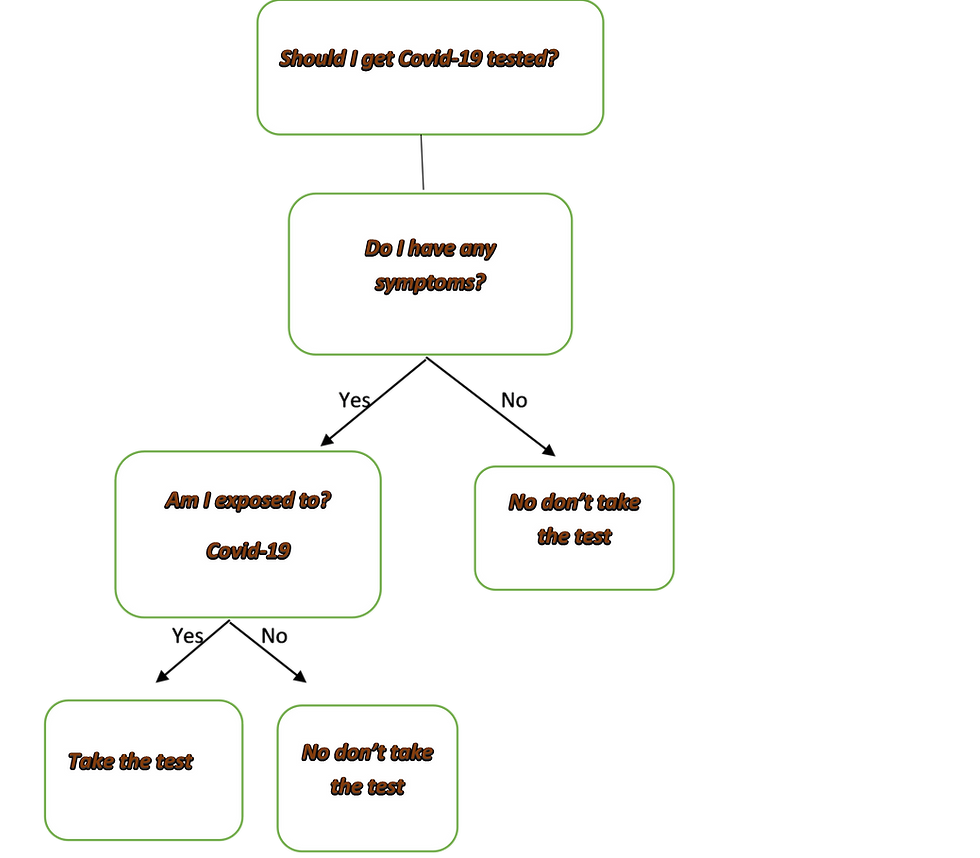top of pageSearch

# A Simple introduction to Decision tree and Support Vector Machines (SVM)Decision tree and Support vector machines are the popular tools used in Machine learning to make predictions. Both these algorithms can be used on classification and regression problems. Without further delay let’s have a short briefing on them…

Decision Tree Making

Decision Trees are a type of Supervised Machine Learning where the data is continuously split according to a certain parameter. The tree can be explained by two entities, namely decision nodes and leaves. The leaves are the decisions or the final outcomes. And the decision nodes are where the data is split.Image by author

An example of a decision tree can be explained using above binary tree. Let’s say we want to predict whether a person needs Covid-19 test using given information’s. The Decision nodes here are questions like ‘Am I exposed’? ‘Do I have symptoms’? And the leaf nodes are outcomes like ‘Take the test’, ‘No don’t take test’. In this case this was a binary classification problem (a yes, no type problem). There are two main types of Decision Trees:

1. Classification trees (Yes/No types)

What we’ve seen above is an example of classification tree, where the outcome was a variable like ‘Take the test’ or ‘No don’t take test’. Here the decision variable is Categorical.

2.Regression trees (Continuous data types)

A regression tree refers to an algorithm where the target variable is and the algorithm is used to predict its value. As an example of a regression type problem, you may want to predict the selling prices of a car, which is a continuous dependent variable. This will depend on both continuous factors like mileage as well as categorical factors like the year its build, accident history and so on.

Strengths and Weakness of Decision Tree The strengths of decision tree methods are:

• Decision trees are easy to understand. Its results are in a set of rules

• It performs classification without requiring much computation.

• It is capable to handle both continuous and categorical variables.

• It provides a clear indication of which fields are most important for prediction or classification.

The weaknesses of decision tree methods:

• Decision trees are less appropriate for estimation tasks where the goal is to predict the value of a continuous attribute.

• They are prone to errors in classification problems with many class and relatively small number of training examples.

• There is a high probability of overfitting in Decision Tree.

Support Vector Machines (SVM):

Support Vector Machines (SVM) is a supervised machine learning algorithm which can be used for classification or regression problems. It uses a technique called the kernel trick to transform your data and then based on these transformations it finds an optimal boundary between the possible outputs. The goal of SVM is to identify an optimal separating hyperplane which maximizes the margin between different classes of the training data.Image data camp

Support Vectors:

Support vectors are the data points, which are closest to the hyperplane. These points will define the separating line better by calculating margins.

Hyperplane:

A hyperplane is a decision plane which separates between a set of objects having different class.

Margin:

A margin is a gap between the two lines on the closest class points.Images data camp

To separate these two classes there are many possible hyperplanes that could be chosen. Our objective is to find the best hyperplane that gives the maximum margin.

Non-Linear Data:

In the above example it was simple to draw a line that separates both the class but it isn’t the same way for all the kind of problems. In such situation the SVM uses Kernel trick to convert the non-separable data into a separable one. For that We will add a third dimension. We create a new z dimension, and that can be calculated a certain way: z = x² + y²Images data camp

SVM Kernel:

A kernel transforms an input data space into the required form. SVM uses a technique called the kernel trick that converts non-separable problem to separable problems by adding more dimension to it. It is most useful in non-linear separation problem. Kernel trick helps you to build a more accurate classifier.

SVMs are helpful text and hypertext categorization, Face Detection, Classification of images, Hand-written characters can be recognized using SVM, Cancer Diagnosis and Prognosis.

Strength and weakness of SVM:

Strength of SVM:

1. It is effective in cases where the number of dimensions is greater than the number of samples.

2. It works really well with a clear margin of separation

3. It uses a subset of training points in the decision function (called support vectors), so it is also memory efficient.

4. It is effective in high dimensional spaces.

Weakness of SVM:

1. It fails to perform well, when we have large data set because the required training time is higher

2. SVM doesn’t directly provide probability estimates

3. It fails to perform, when the data set has more noise.

Conclusion:

There is no perfect model, each of the model is a tool. Meaning that it needs to be properly justified and optimized in accordance to each of the case.